# How to implement Radix sort algorithm in JavaScript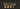In this tutorial, we are going to learn about Radix sort algorithm and its implementation in JavaScript.

## What is Radix sort ?

Radix sort is a non-comparison based sorting algorithm where it grouping by the number place and position.

Example : [4, 57, 7, 3, 933]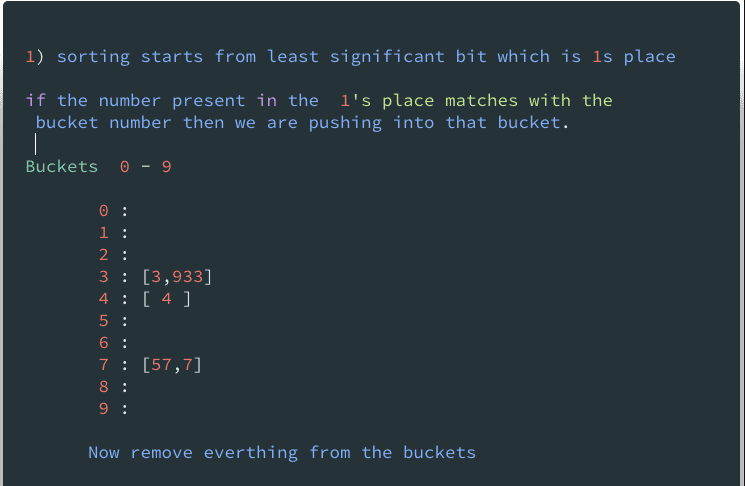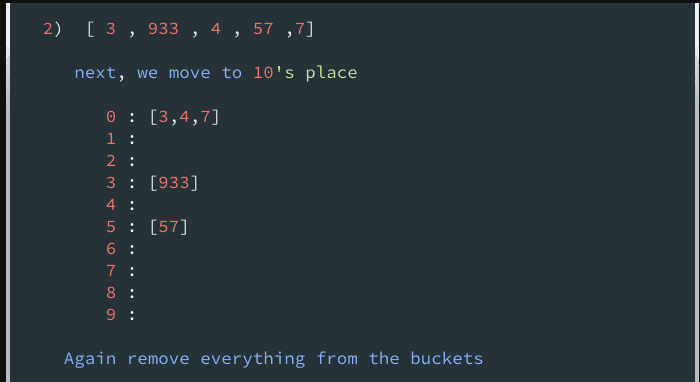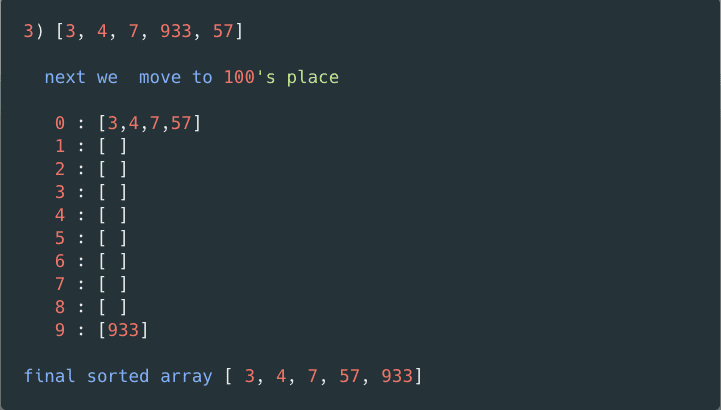First, we are implementing some helper functions

1. If we pass an array of numbers `getMax` function returns back max number length.

suppose `getMax([3, 44, 533]) // output 3`

``````function getMax(arr) {

let max = 0;
for (let num of arr) {
if (max < num.toString().length) {
max = num.toString().length
}
}
return max
}``````

### Second helper function

if we pass a number and place `getPosition` function returns back number in that place.

`````` function getPosition(num, place){
return  Math.floor(Math.abs(num)/Math.pow(10,place))% 10
}``````

suppose

``````         getPosition(243 , 1 )  // 4
getPosition(123,  0)   // 3
getPosition(943, 2)   // 9``````

Main algorithm starts.

`````` function radixSort(arr){

const max = getMax(arr); // returns length  of max digit

return arr
}``````

If our max length is 4 we only loop 4 times

next, we need to write two for loops

first for loop helps us to get the number of places like 1’s, 10’s, 100’s and resetting the buckets.

`````` function radixSort(arr){

const max = getMax(arr);

for(let i=0;i<max;i++){
let buckets = Array.from({length:10},()=>[ ]) // creating 10 empty arrays
arr = [].concat(...buckets);
}
return arr
}``````

second for loop is used to loop over every number in the unsorted array and push into it’s desired buckets.

``````function radixSort(arr) {

const max = getMax(arr); // length of the max digit in the array

for (let i = 0; i < max; i++) {
let buckets = Array.from({ length: 10 }, () => [ ])
for (let j = 0; j < arr.length; j++) {
buckets[getPosition(arr[ j ], i)].push(arr[ j ]); // pushing into buckets
}
arr = [ ].concat(...buckets);
}
return arr
}

console.log(radixSort([4, 57, 7, 3, 933])) // [3,4,7,57,933]``````

Algorithm is Tested using Mocha and chai

### Radix sort Time and space complexity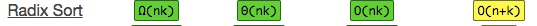• n - number of the elements in the array
• k - Max number length or word size

## Top Udemy Courses##### JavaScript - The Complete Guide 2020 (Beginner + Advanced)
45,614 students enrolled
52 hours of video content
View Course##### React - The Complete Guide (incl Hooks, React Router, Redux)
284,472 students enrolled
40 hours of video content
View Course##### Vue - The Complete Guide (w/ Router, Vuex, Composition API)
130,921 students enrolled
48.5 hours of video content
View Course